# AP Board 9th Class Maths Solutions Chapter 4 Lines and Angles Ex 4.1

AP State Syllabus AP Board 9th Class Maths Solutions Chapter 4 Lines and Angles Ex 4.1 Textbook Questions and Answers.

## AP State Syllabus 9th Class Maths Solutions 4th Lesson Lines and Angles Exercise 4.1

Question 1.
In the given figure, name: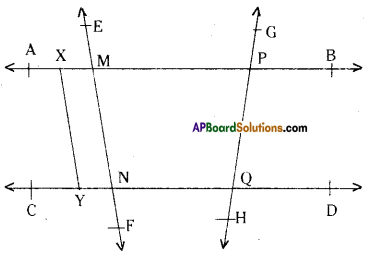i) Any six points
Solution:
A, B, C, D, P, Q, M, N etc.ii) Any five line segments
Solution:
$$\overline{\mathrm{AX}}, \overline{\mathrm{XM}}, \overline{\mathrm{MP}}, \overline{\mathrm{PB}}, \overline{\mathrm{MN}}, \overline{\mathrm{PQ}}, \overline{\mathrm{AB}} \ldots \ldots$$ etc.

iii) Any four rays
Solution:
$$\overline{\mathrm{MA}}, \overline{\mathrm{PA}}, \overline{\mathrm{PB}}, \overline{\mathrm{NC}}, \overline{\mathrm{QD}} \ldots \ldots$$ etc.

iv) Any four lines
Solution:
$$\overline{\mathrm{MA}}, \overline{\mathrm{PA}}, \overline{\mathrm{PB}}, \overline{\mathrm{NC}}, \overline{\mathrm{QD}} \ldots \ldots$$

v) Any four collinear points
Solution:
A, X, M, P and B are collinear points on the line $$\overline{\mathrm{AB}}$$.

Question 2.
Observe the following figures and identify the type of angles in them.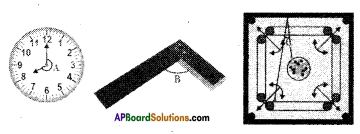Solution:
∠A – reflex angle
∠B – right angle
∠C – acute angleQuestion 3.
State whether the following state¬ments are true or false
i) A ray has no end point.
ii) Line $$\overline{\mathrm{AB}}$$ is the same as line $$\overline{\mathrm{BA}}$$
iii) A ray $$\overline{\mathrm{AB}}$$ is same as the ray $$\overline{\mathrm{BA}}$$
iv) A line has a definite length.
v) A plane, has length and breadth but no thickness.
vii) Two lines may intersect in two points.
viii) Two intersecting lines cannot both be parallel to the same line.
Solution:
i) A ray has no end point. – False
ii) Line $$\overline{\mathrm{AB}}$$ is the same as line $$\overline{\mathrm{BA}}$$ – True
iii) A ray $$\overline{\mathrm{AB}}$$ is same as the ray $$\overline{\mathrm{BA}}$$ – False
iv) A line has a definite length. – False
v) A plane, has length and breadth but no thickness. – True
vi) Two distinct points always determine a unique line. – True
vii) Two lines may intersect in two points. – False
viii) Two intersecting lines cannot both be parallel to the same line. – TrueQuestion 4.
What is the angle between two hands of a clock when the lime in the clock
is
a) 9 ‘o clock
b) 6 ‘o clock
c) 7 ; 00 p.m.
Solution: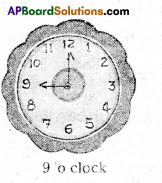a) 12 hours = = 360°
1 hour = $$\frac{360^{\circ}}{12}$$ = 30°
∴Angle between hands when the time is 9 o clock = 3 x 30 = 90

b)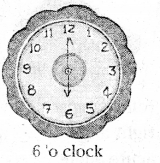Angle between hands = 6 x 30° = 180°c)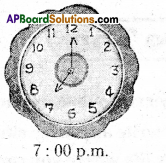Angle between hands = 7 x 30° = 210°